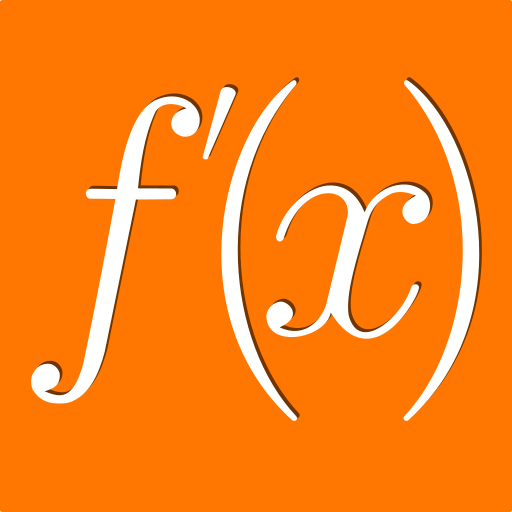# Derivatives

4.8★

Android

### Images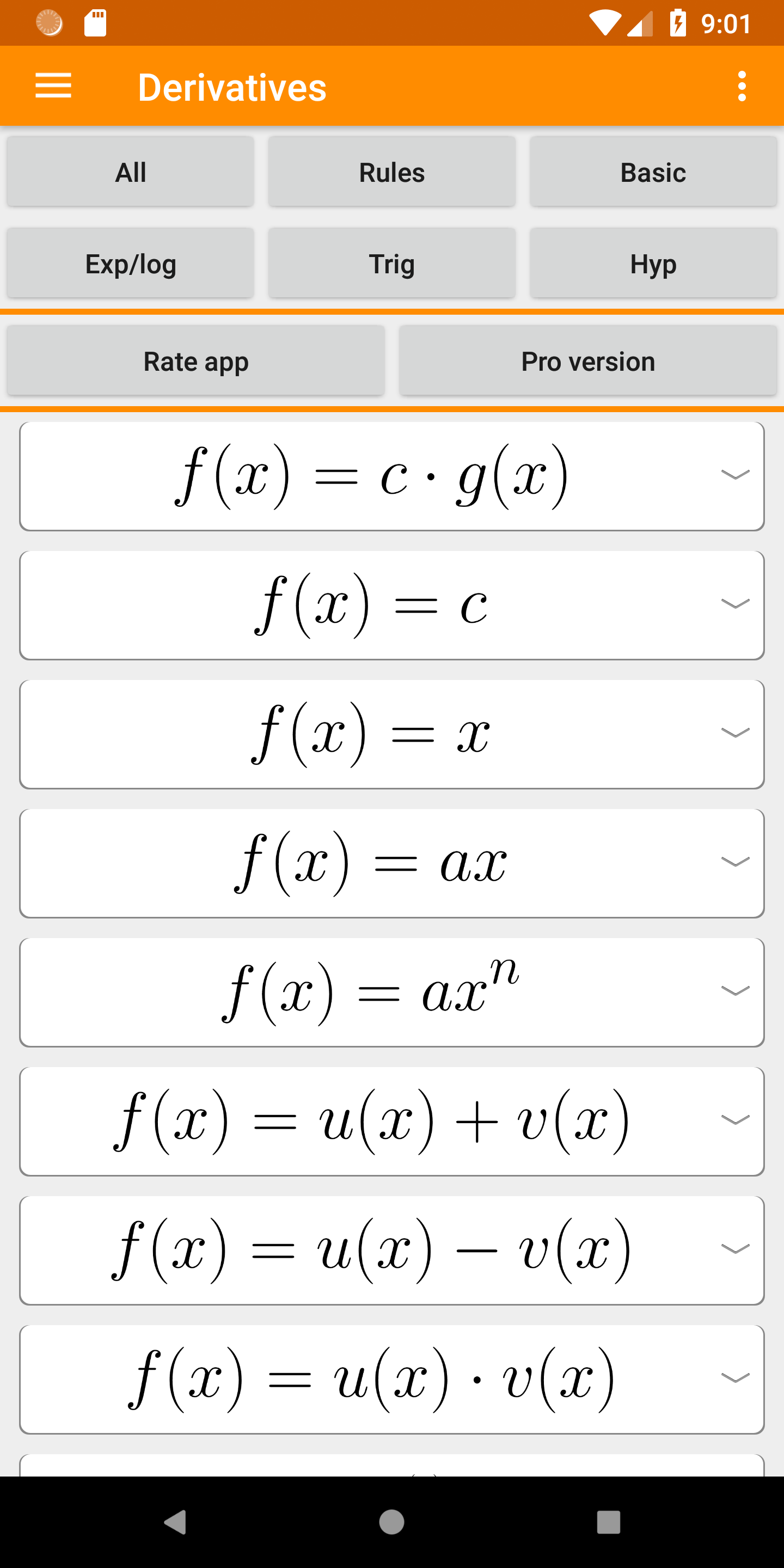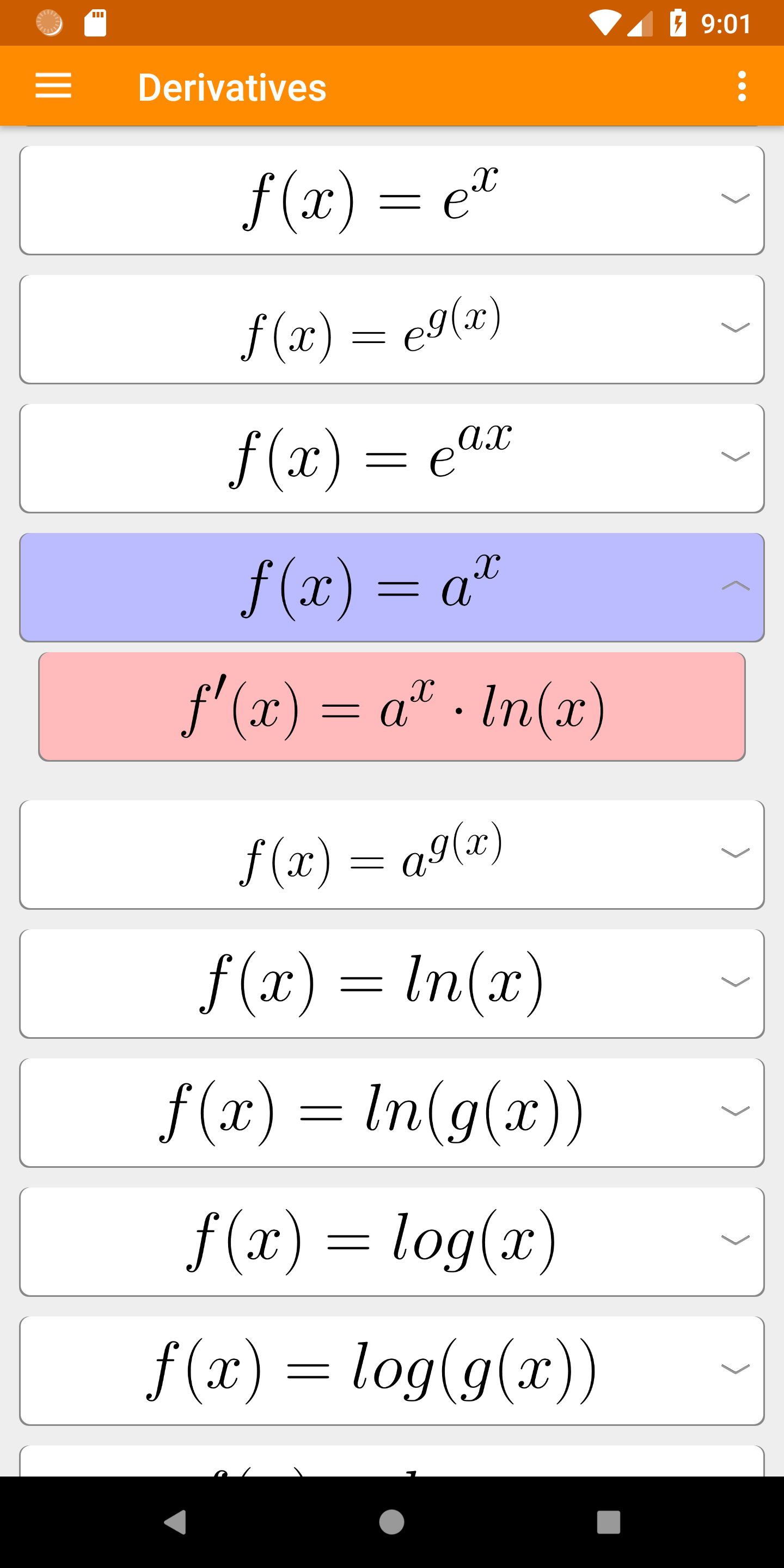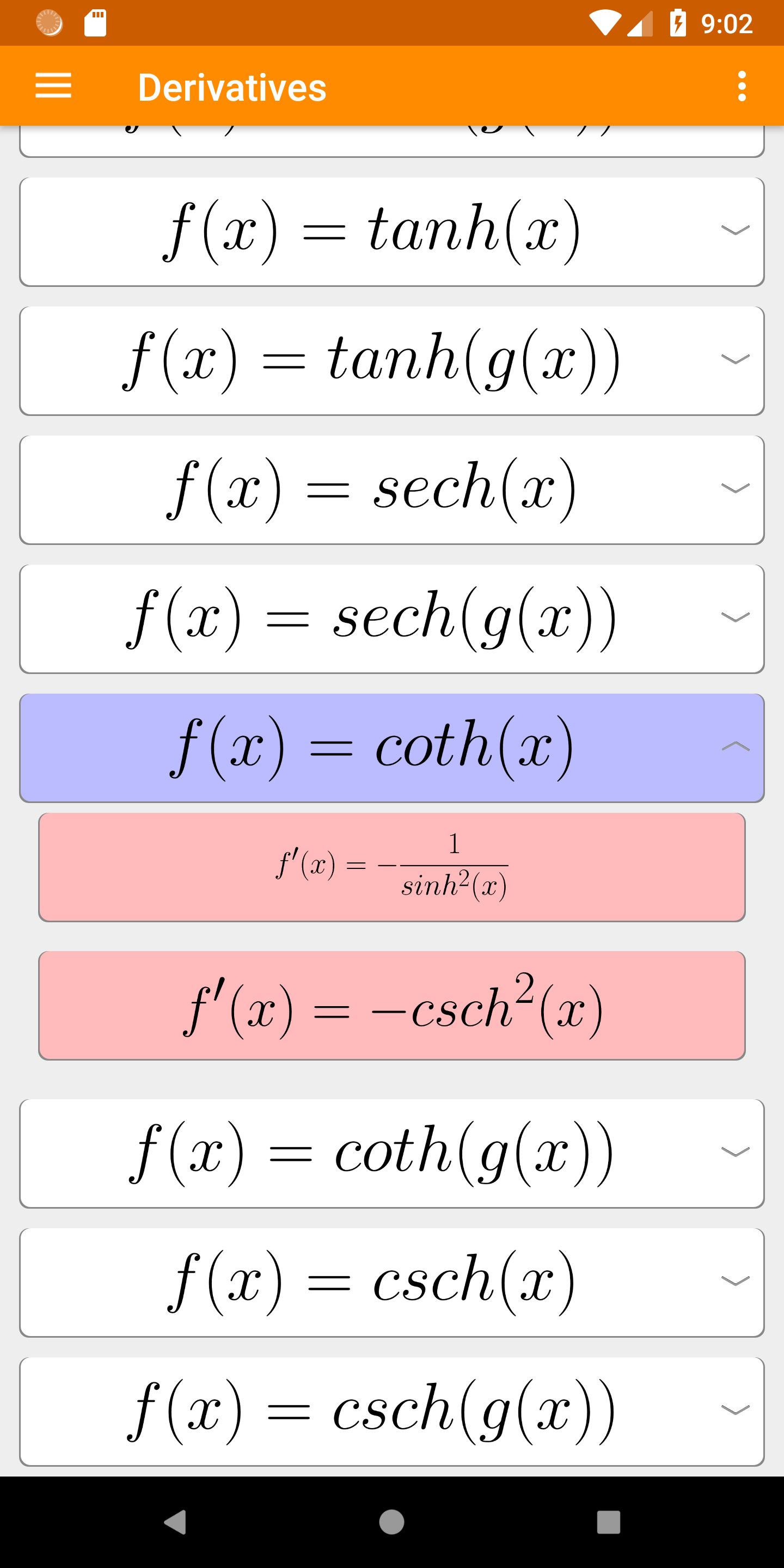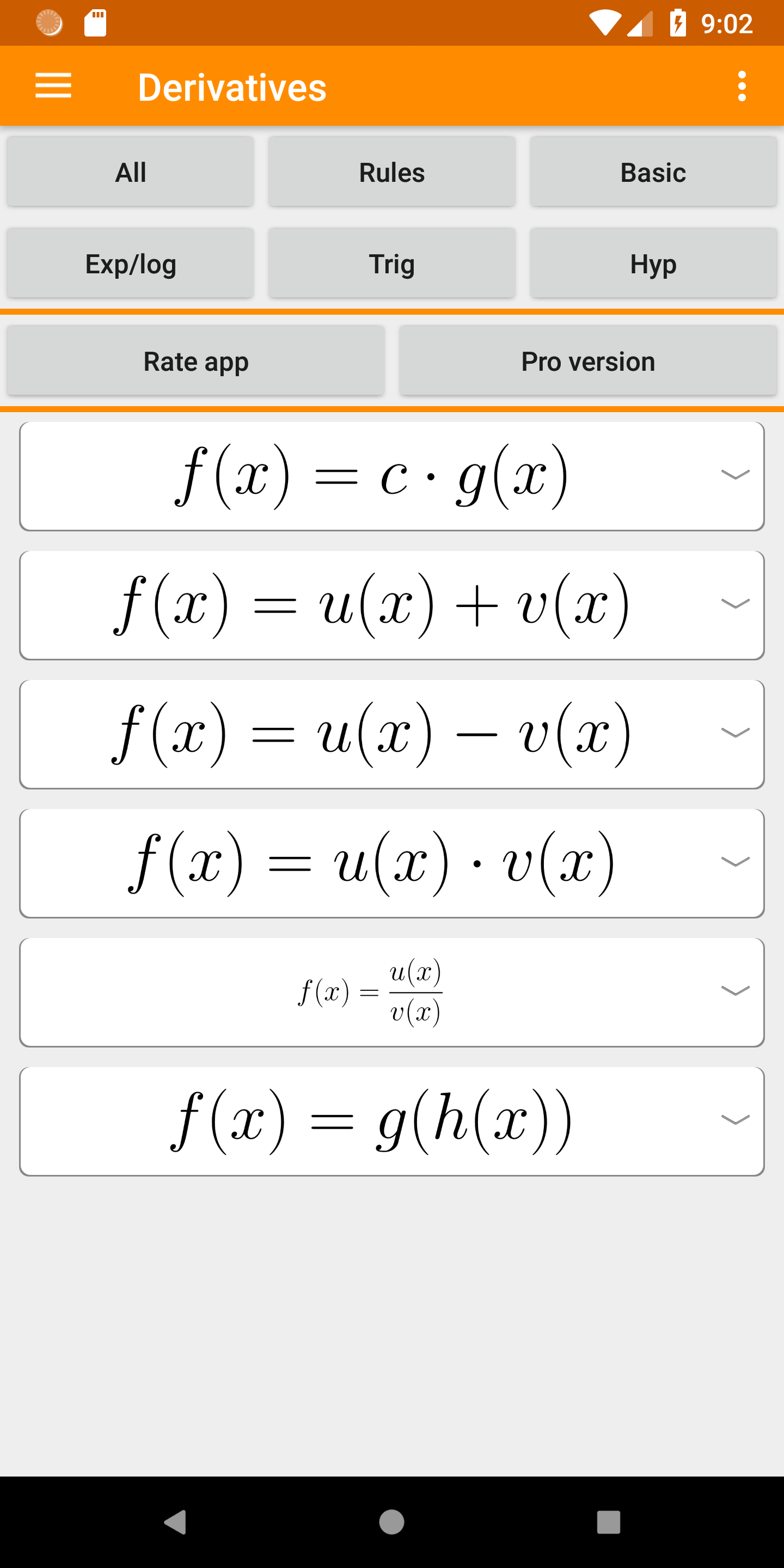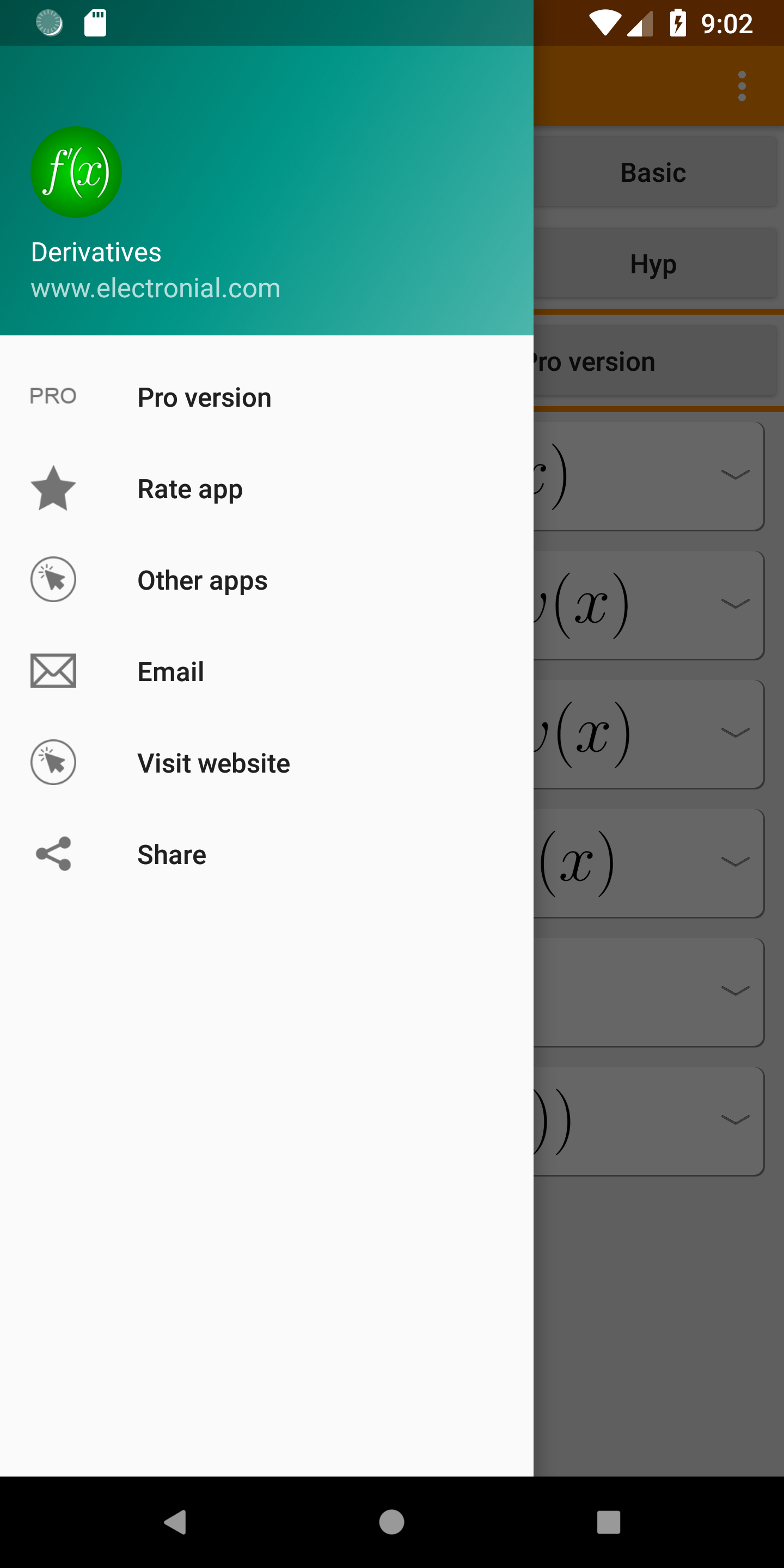### Description

This app contains all common derivative functions.
The app is free and easy to use
Formula types:
• Derivation rules
• Basic functions
• Exponential functions
• Logarithmic functions
• Trigonometric functions
• Hyperbolic functions